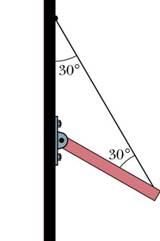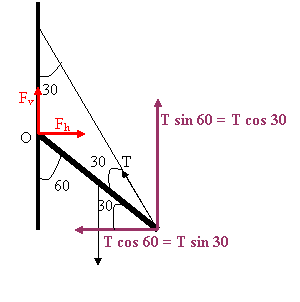Example 13.4.2

One end of uniform beam that weighs 222 N is attached to a wall with a hinge. The other end is supported bt a wire. Find the tension in the wire.The beam is in equilibrium, the sum of the forces and the sum of the torques acting on it both vanish. As we see in the figure, the beam makes an angle of 60 with the vertical and the wire makes an angle of 30 with the vertical.We calculate the torques around the hinge. Their sum is:

T L sin 30 - W (L/2) sin 60 = 0

Here W is the force of gravity acting at the center of the beam, and T is the tension force of the wire. We solve for the tension to get:

T = W sin 60 / (2 sin 30) = (222 N) sin 60 / (2 sin 30) = 192.3 N .